Next: Internal Energy Up: Classical Thermodynamics Previous: Isothermal Atmosphere

Of course, we know that the atmosphere is not isothermal. In fact, air temperature falls quite noticeably with increasing altitude. In ski resorts, the general rule of thumb is that the temperature drops by about 1 degree per 100 meters increase in altitude. Many people cannot understand why the atmosphere gets colder with increasing height. They reason that because higher altitudes are closer to the Sun they ought to be hotter. In fact, the explanation is quite simple. It depends on three important properties of air. The first property is that air is transparent to most, but by no means all, of the electromagnetic spectrum. In particular, most infrared radiation, which carries heat energy, passes straight through the lower atmosphere, and heats the ground. In other words, the lower atmosphere is heated from below, not from above. The second important property of air is that it is constantly in motion. In fact, the lower 20 kilometers of the atmosphere (the so-called troposphere) are fairly thoroughly mixed. You might think that this would imply that the atmosphere is isothermal. However, this is not the case because of the final important property of air: namely, it is a very poor conductor of heat. This, of course, is why woolly sweaters work--they trap a layer of air close to the body, and, because air is such a poor conductor of heat, you stay warm.

Imagine a packet of air that is swirling around in the atmosphere. We would expect it to always remain at the same pressure as its surroundings, otherwise it would be mechanically unstable. It is also plausible that the packet moves around too quickly to effectively exchange heat with its surroundings, because air is very a poor heat conductor, and heat flow is consequently quite a slow process. So, to a first approximation, the air in the packet is adiabatic. In a steady-state atmosphere, we expect that, as the packet moves upwards, expands due to the reduced pressure, and cools adiabatically, its temperature always remains the same as that of its immediate surroundings. This means that we can use the adiabatic gas law to characterize the cooling of the atmosphere with increasing altitude. In this particular case, the most useful manifestation of the adiabatic law is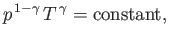(6.70)

giving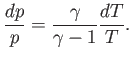(6.71)

Combining the previous expression with the equation of hydrostatic equilibrium, (6.67), we obtain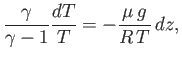(6.72)

or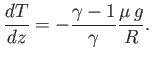(6.73)

Now, the ratio of specific heats for air (which is effectively a diatomic gas) is about 1.4. (See Table 6.1.) Hence, we can deduce, from the previous expression, that the temperature of the atmosphere decreases with increasing height at a constant rate of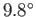C per kilometer. This value is called the (dry) adiabatic lapse rate of the atmosphere. Our calculation accords well with the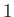degree colder per 100 meters higher'' rule of thumb used in ski resorts. The basic reason that air is colder at higher altitudes is that it expands as its pressure decreases with height. It, therefore, does work on its environment, without absorbing any heat (because of its low thermal conductivity), so its internal energy, and, hence, its temperature decreases.

According to the adiabatic lapse rate calculated previously, the air temperature at the cruising altitude of airliners (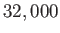feet) should be about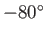C (assuming a sea level temperature of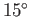C). In fact, this is somewhat of an underestimate. A more realistic value is about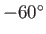C. The explanation for this discrepancy is the presence of water vapor in the atmosphere. As air rises, expands, and cools, water vapor condenses out, releasing latent heat, which prevents the temperature from falling as rapidly with height as the adiabatic lapse rate would predict. (See Exercise 6.) In fact, in the tropics, where the air humidity is very high, the lapse rate of the atmosphere (i.e., the rate of decrease of temperature with altitude) is significantly less than the adiabatic value. The adiabatic lapse rate is only observed when the humidity is low. This is the case in deserts, in the arctic (where water vapor is frozen out of the atmosphere), and, of course, in ski resorts.

Suppose that the lapse rate of the atmosphere differs from the adiabatic value. Let us ignore the complication of water vapor, and assume that the atmosphere is dry. Consider a packet of air that moves slightly upwards from its equilibrium height. The temperature of the packet will decrease with altitude according to the adiabatic lapse rate, because its expansion is adiabatic. We shall assume that the packet always maintains pressure balance with its surroundings. It follows that because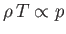, according to the ideal gas law,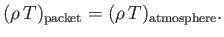(6.74)

If the atmospheric lapse rate is less than the adiabatic value then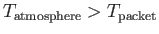implying that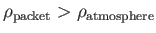. So, the packet will be denser than its immediate surroundings, and will, therefore, tend to fall back to its original height. Clearly, an atmosphere whose lapse rate is less than the adiabatic value is vertically stable. On the other hand, if the atmospheric lapse rate exceeds the adiabatic value then, after rising a little way, the packet will be less dense than its immediate surroundings, and will, therefore, continue to rise due to buoyancy effects. Clearly, an atmosphere whose lapse rate is greater than the adiabatic value is vertically unstable. This effect is of great importance in meteorology. The normal stable state of the atmosphere is for the lapse rate to be slightly less than the adiabatic value. Occasionally, however, the lapse rate exceeds the adiabatic value, and this is always associated with extremely disturbed weather patterns.

Let us consider the temperature, pressure, and density profiles in an adiabatic atmosphere. We can directly integrate Equation (6.73) to give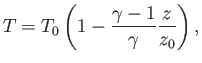(6.75)

where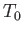is the ground-level temperature, and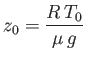(6.76)

the isothermal scale-height calculated using this temperature. The pressure profile is easily calculated from the adiabatic gas law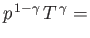constant, or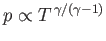. It follows that(6.77)

Consider the limit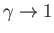. In this limit, Equation (6.75) yieldsindependent of height (i.e., the atmosphere becomes isothermal). We can evaluate Equation (6.77) in the limit asusing the mathematical identity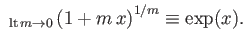(6.78)

We obtain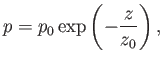(6.79)

which, not surprisingly, is the predicted pressure variation in an isothermal atmosphere. In reality, the ratio of specific heats of the atmosphere is not unity, but is about 1.4 (i.e., the ratio for diatomic gases), which implies that in the real atmosphere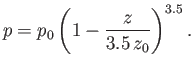(6.80)

In fact, this formula gives very similar results to the isothermal formula, Equation (6.79), for heights below one scale-height (i.e.,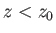). For heights above one scale-height, the isothermal formula tends to predict too high a pressure. (See Figure 6.1.) So, in an adiabatic atmosphere, the pressure falls off more quickly with altitude than in an isothermal atmosphere, but this effect is only noticeable at pressures significantly below one atmosphere. In fact, the isothermal formula is a fairly good approximation below altitudes of about 10 kilometers. Because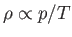, the variation of density with height is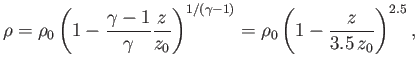(6.81)

where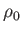is the density at ground level. Thus, the density falls off more rapidly with altitude than the temperature, but less rapidly than the pressure.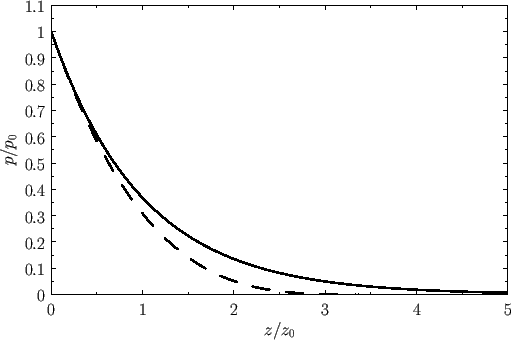Note that an adiabatic atmosphere has a sharp upper boundary. Above height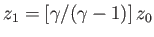, the temperature, pressure, and density are all zero. In other words, there is no atmosphere. For real air, with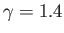, the upper boundary of an adiabatic atmosphere lies at height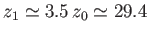kilometers above sea level. This behavior is quite different to that of an isothermal atmosphere, which has a diffuse upper boundary. In reality, there is no sharp upper boundary to the atmosphere. The adiabatic gas law does not apply above about 20 kilometers (i.e., in the stratosphere) because, at these altitudes, the air is no longer strongly mixed. Thus, in the stratosphere, the pressure falls off exponentially with increasing height.

In conclusion, we have demonstrated that the temperature of the lower atmosphere should decrease approximately linearly with increasing height above ground level, while the pressure should decrease far more rapidly than the temperature, and the density should decrease at some intermediate rate. We have also shown that the lapse rate of the temperature should be about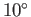C per kilometer in dry air, but somewhat less than this in wet air. In fact, all of these predictions are, more or less, correct. It is amazing that such accurate predictions can be obtained from the two simple laws--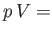constant for an isothermal gas, and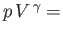constant for an adiabatic gas.Next: Internal Energy Up: Classical Thermodynamics Previous: Isothermal Atmosphere
Richard Fitzpatrick 2016-01-25